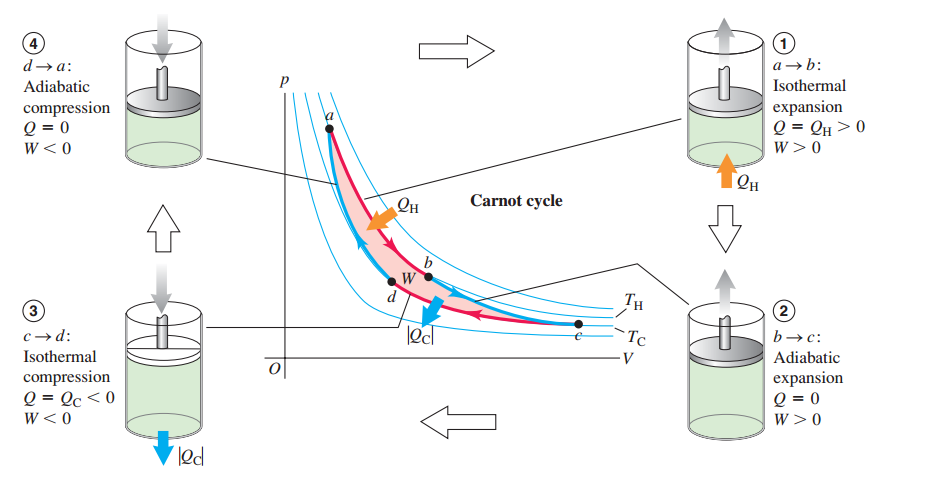# Thermodynamics

Two moles of an ideal gas at temperature $300 \text{ K}$ was cooled isochorically so that the pressure was reduced to half. Then in an isobaric process the gas expanded till the temperature got back to initial value.

Find the amount of heat absorbed by the gas in the entire process.

Consider the value of gas constant to be $8.3 \dfrac { \text J }{ \text{mol}\times \text K }$.×